Courses

# Orbital Angular Momentum, Hydrogen Atom (Part - 2) - Angular Momentum, CSIR-NET Physical Sciences Physics Notes | EduRev

## Physics : Orbital Angular Momentum, Hydrogen Atom (Part - 2) - Angular Momentum, CSIR-NET Physical Sciences Physics Notes | EduRev

The document Orbital Angular Momentum, Hydrogen Atom (Part - 2) - Angular Momentum, CSIR-NET Physical Sciences Physics Notes | EduRev is a part of the Physics Course Physics for IIT JAM, UGC - NET, CSIR NET.
All you need of Physics at this link: Physics

Schr¨odinger equation in three dimensions, central potential

The knowledge we gained on angular momentum is particularly useful when treating real life problems. As our world is three dimensional, we need to generalize the treatment of Schro¨dinger equation to 3-d.
The time-independent Schro¨dinger equation becomes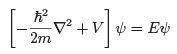(56)

where, in 3-d,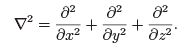(57)

In many problems in physics, the potential is central, namely, V = V (r); this means that the potential is spherically symmetric, and is not a function of θ or φ. In this type of systems - the best representative may be the hydrogen atom to be discussed shortly, it is best to work in spherical coordinates, r, θ, φ.

In spherical coordinates, the laplacian becomes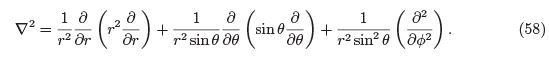Comparing to Equation 17, we see that the last two terms of the laplacian are equal to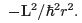Thus, we can write the Hamiltonian as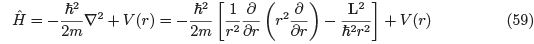and the time-independent Schro¨dinger equation is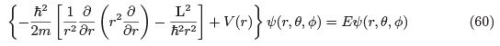In order to proceed, we note that all of the angular momentum operators: Lx, Ly , Lz and Ldo not operate on the radial variable, r; this can be seen directly by their description in spherical coordinates, equation 17. This means that all these operators commute with V (r): [Lz , V (r)] = 0, etc. Furthermore, since Lx, Ly and Lz commute with L2, we conclude that all of the angular momentum operators commute with the Hamiltonian,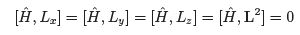This means that it is possible to obtain solutions to Schro¨dinger equation (Equation 60) which are common eigenfunctions of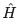, L2 and Lz .

We already know simultaneous eigenfunctions of L2 and Lz : these are of course the spherical harmonics, Ylm(θ, φ). Thus, a full solution to Schro¨dinger equation can be written as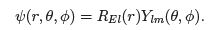(62)

REl (r) is a radial function of r, which we need to ﬁnd. The subscripts E and l mark the fact that in general, we obtain diﬀerent functions for diﬀerent values of the energy(E ) and the orbital angular momentum quantum number l. It is independent, though, on the magnetic quantum number m, as can be seen by inserting this solution into Schro¨dinger equation (in which the operator L2 appears explicitly, but not Lz ).

We may put the solution in Equation (62) in Schro¨dinger equation (60), and use the fact that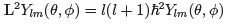(Equation 25), to obtain an equation for REl (r),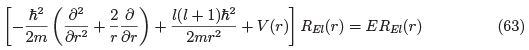To be physically acceptable, the wave functions must be square integrable, and normalized to 1: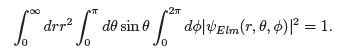(64)

.We already know that the spherical part, Ylm(θ, φ) is normalized; see Equation 42. Thus, the radial part of the eigenfunctions must satisfy the normalization condition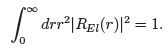(65)

We may further simplify Equation 63 by changing a variable,

uEl (r) = rREl (r) (66)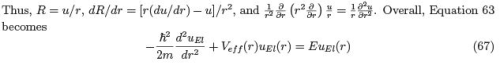where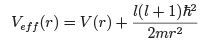(68)
is an eﬀective potential; in addition to the interaction potential, V (r) it contains a repulsive centrifugal barrier,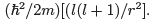With the inclusion of this potential, Equation 67 has an identical form to the 1-d (timeindependent) Schro¨dinger equation. The only diﬀerence is that it is physically meaningful only for r > 0, and we must provide the boundary condition at r = 0. The boundary conditions are provided by the physical requirement that the function REl (r) remains ﬁnite at the origin, r = 0. Since REl (r) = uEl (r)/r, this implies

uEl (0) = 0. (69)

The hydrogen atom

Perhaps the most important demonstration of the above analysis (and of quantum mechanics in general) is the ability to predict the energy levels and wave functions of the hydrogen atom. This is the simplest atom, that contains one proton and one electron. The proton is heavy (mp/me = 1836) and is essentially motionless - we can assume it being at the origin, r = 0). The proton has a positive charge +q, and the electron a negative charge, −q. We can therefore use Coulumb’s law to calculate the potential energy: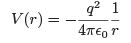(70)

(in SI units).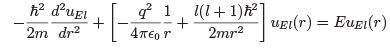(71)

Before proceeding to solve this equation, we note the following. At r → ∞, Vef f (r) → 0.
This means that for any value of positive energy (E > 0), one could ﬁnd an acceptable eigenfunction uEl (r). Therefore, there is a continuous spectrum for E > 0, describing scattering between electron and proton (this will be dealt with in next year’s QM...). We focus here on solutions for which E < 0. These are called bound states.
We proceed by some change of variables: We write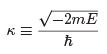(72)

(note that E < 0, and so κ is real). Equation 71 becomes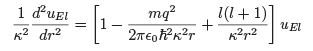(73)

We next introduce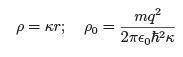(74)
and write Equation 73 as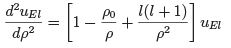(75)

We proceed along lines which are somewhat similar to those taken in deriving the SHO.
We begin by examining the asymptotic behavior. We note that when ρ → ∞, Equation 75 is approximately d2uEl /dρ2 ≈ uEl , which admits the general solution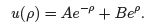However, in order for uEl to remain ﬁnite as ρ → ∞, we must demand B = 0, implying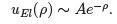On the other hand, as ρ → 0, the centrifugal term dominates (apart when l = 0, but, as will be seen, the result is valid there too), and one can write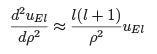with the general solution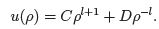Again, the second term, ρ−l diverges as ρ → 0, implying that we must demand D = 0. Thus,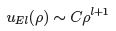for small ρ.
This discussion motivates another change of variables, writing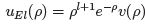(76)

where v(ρ) ≡ vEl (ρ), and the subscripts E l are omitted for clarity.
With this change of variables, we have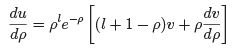and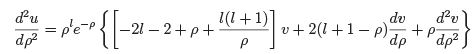and Equation 75 becomes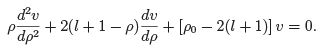(77)

We search for solution v(ρ) is terms of power series: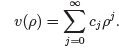(78)

We can thus write: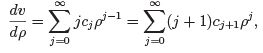(79)

and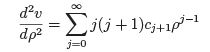(80)

similar to the analysis of the SHO, we insert these results into Equation 77, and equate the coeﬃcients of each individual power law of ρ to write:Similar to the SHO case, we note that for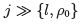we have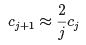which gives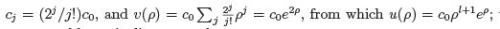this of course is unacceptable, as it diverges at large ρ.

This means that the series must terminate, namely there is a maximum integer, jmax for which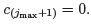. From Equation 81 this gives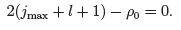(82)

We can now deﬁne the principle quantum number, n via n ≡ jmax + l + 1. (83)

Thus, ρ0 = 2n. But ρdetermines the energy via equations 72 and 74: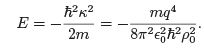(84)

We therefore conclude that the allowed energies are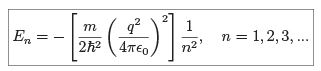(85)

This is the well-known Bohr’s formula.
Using again equation 74, one ﬁnds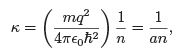(86)

where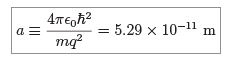(87)

is known as the Bohr’s radius.
The ground state (namely, the state of lowest energy) is obtained by putting n = 1 in Equation 85. Putting the values of the physical constants, one ﬁnds that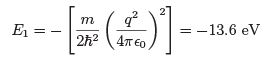(88)

This is the binding energy of the hydrogen atom - the amount of energy one needs to give to the electron in the hydrogen atom that is in its ground state to ionize the atom (=release the electron).
Furthermore, E2 = E1/22 = E1/4 = −3.4 eV, etc.

The wavefunctions

Returning to Equation 62, the wavefunctions are given by

ψ(r, θ, φ) = REl (r)Ylm(θ, φ).

where (using Equations 66 and 76)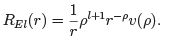(89)

Here, v(ρ) is given by the polynomial of degree jmax = n − l − 1 (see Equation 83).

In the ground state, n = 1; Equation 83 forces l = 0 and jmax = 0. Since l = 0, we known that m = 0 as well (see Equation 35). This means that the wave function is given by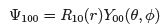(90)

Using the recursion formula (Equation 81), with j = 0, leads to c1 = 0; that is, v(ρ) = 0 is simply a constant. This implies that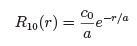(91)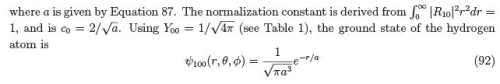The next energy level is n = 2, which represents the ﬁrst excited state. There are, in fact four diﬀerent states with this same energy: one state with l = 0, in which case also m = 0; and l = 1, in which case m = −1, 0, +1. For l = 0, Equation 81 gives c1 = −c0, c2 = 0, namely v(ρ) = c0(1 − ρ). This implies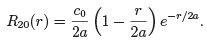(93)

For l = 1, the recursion formula terminates the series after a single term, v(ρ) is constant, and one ﬁnds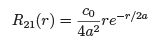(94)
and so on.
In fact, one can write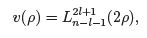(95)
where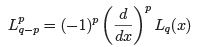(96)

is an associated Laguerre polynomial, and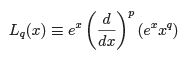(97)

is known as the qth Laguerre polynomial. I list in table 5.1 the ﬁrst few radial eigenfunctions of the hydrogen.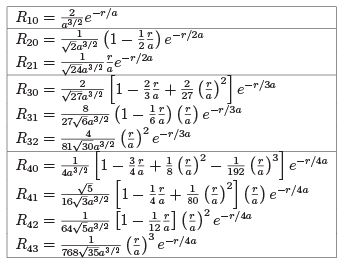Table 2: The ﬁrst few radial wavefunctions for the hydrogen, REl (r).
According to the standard interpretation of the wavefunction, the quantity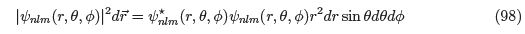represents the probability of ﬁnding the electron in the volume element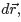, when the system is in the stationary state speciﬁed by the quantum numbers (n, l, m).

Since ψ(r, θ, φ) = REl (r)Ylm(θ, φ) (see Equation 62), the position probability density |ψnlm(r, θ, φ)|2 is composed of a radial part that depends only on r, and an angular part that depends only on θ (recall that the dependence on φ disappears).

We can write the radial part as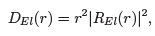(99)

which is known as the radial distribution function. In ﬁgure 2 I plot the ﬁrst few radial functions REl and the radial distribution function.

Finally, in ﬁgures 3 – 5 I give a few examples of the full probability density of ﬁnding the electron in (r, θ) for a hydrogen atom, namely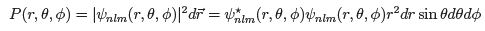(100)

for few values of the quantum numbers n, l, and m. As is obvious from the discussion above, this probability is independent on φ, but only on r and θ.

As a ﬁnal remark, I would add that in the usual spectroscopic notation the quantum number l is replaced by a letter, according to the following table: Thus, the energy levels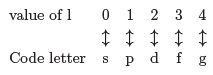are denoted by two symbols: the ﬁrst is the principal quantum number n, and the second is a letter corresponding to l.
The ground state (n = 1) is denoted by 1s; The ﬁrst excited state (n = 2) contains one 2s state, and three 2p state, corresponding to m = −1, 0, +1 - so total 4 states; the second excited state contains one 3s state, three 3p states and ﬁve 3d states, with m = −2, −1, 0, +1, +2, so total of 9 states; etc.

Offer running on EduRev: Apply code STAYHOME200 to get INR 200 off on our premium plan EduRev Infinity!

159 docs

,

,

,

,

,

,

,

,

,

,

,

,

,

,

,

,

,

,

,

,

,

,

,

,

,

,

,

;